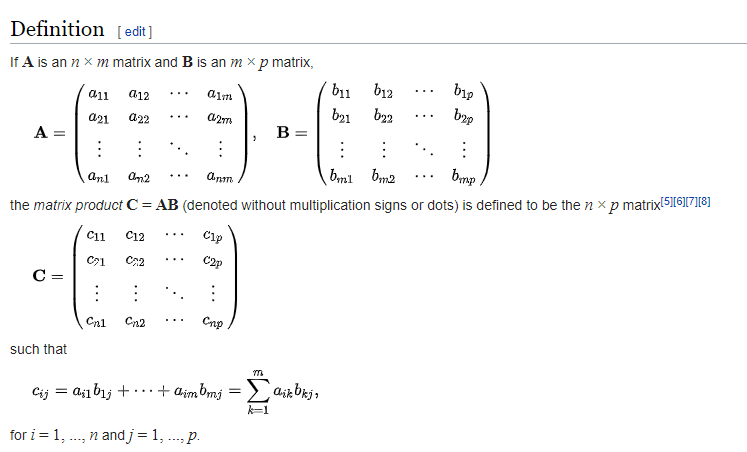n1try/matmult.go Last active Dec 4, 2018
 Hi, your program is not correct... Your program would output [[1 2 3] [4 5 6]] * [[0.5 0.5] [0.2 0.8] [0.7 0.3]] = [[0.5 0.4 2.1] [2 4 1.8000001]] The size of the resulting matrix is not even right. A 2x3 matrix times a 3x2 matrix should give you a 2x2 matrix, while yours gives back a 2x3 matrix. The correct code really should be package main import ( "errors" "fmt" ) func main() { X := [][]float32{ []float32{1.0, 2.0, 3.0}, []float32{4.0, 5.0, 6.0}, } w := [][]float32{ []float32{0.5, 0.2, 0.7}, []float32{0.5, 0.8, 0.3}, } out, _ := multiply(X, transpose(w)) fmt.Println(out) } func transpose(x [][]float32) [][]float32 { out := make([][]float32, len(x)) for i := 0; i < len(x); i += 1 { for j := 0; j < len(x); j += 1 { out[j] = append(out[j], x[i][j]) } } return out } func multiply(x, y [][]float32) ([][]float32, error) { if len(x) != len(y) { return nil, errors.New("Can't do matrix multiplication.") } out := make([][]float32, len(x)) for i := 0; i < len(x); i++ { out[i] = make([]float32, len(y)) for j := 0; j < len(y); j++ { for k := 0; k < len(y); k++ { out[i][j] += x[i][k] * y[k][j] } } } return out, nil } You can test it in https://play.golang.org/p/uVJLR8qAxv3 Notice there are 3 loops, which is also confirmed in an implementation in python (https://www.programiz.com/python-programming/examples/multiply-matrix). A more mathy version can be found here: (https://en.wikipedia.org/wiki/Matrix_multiplication)